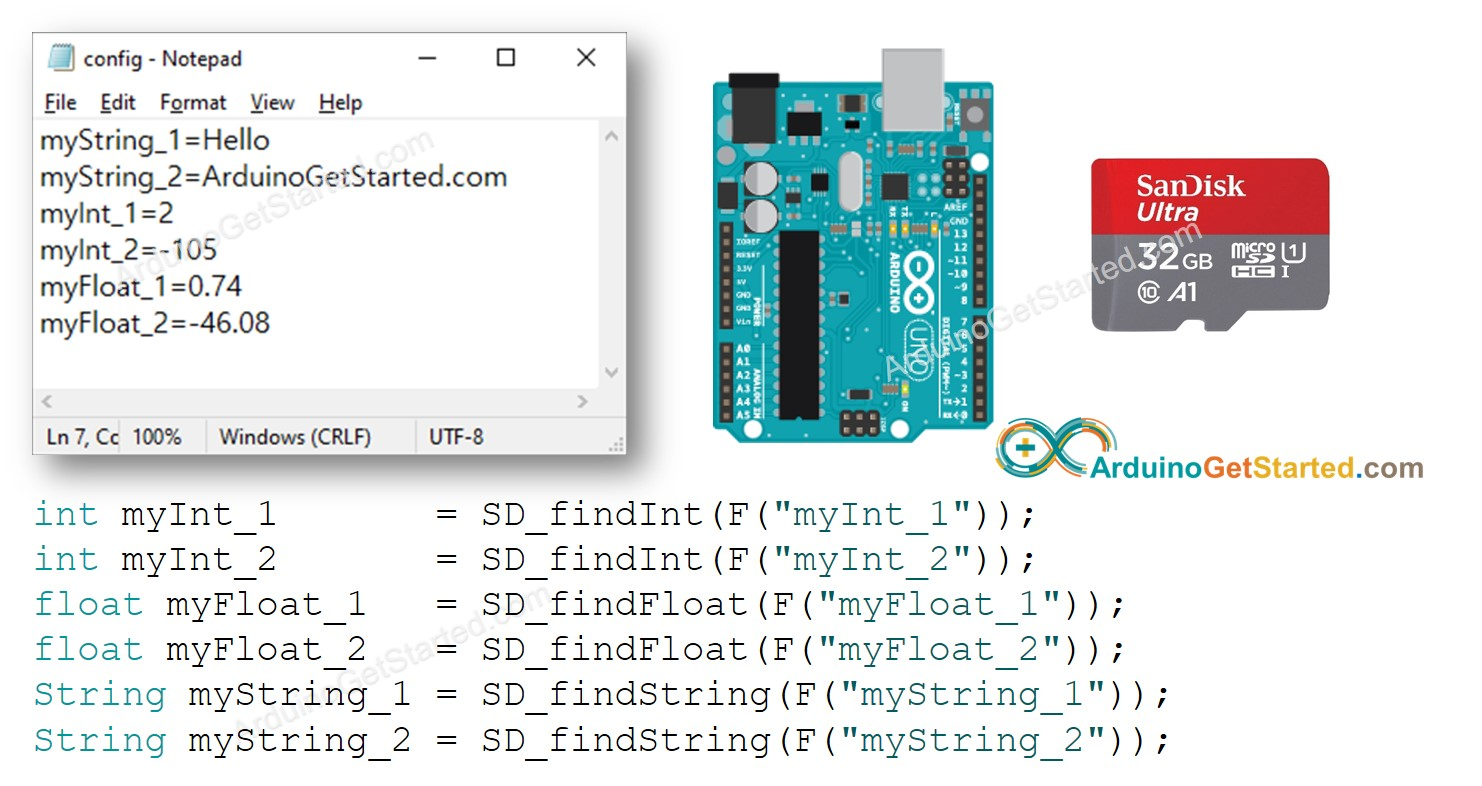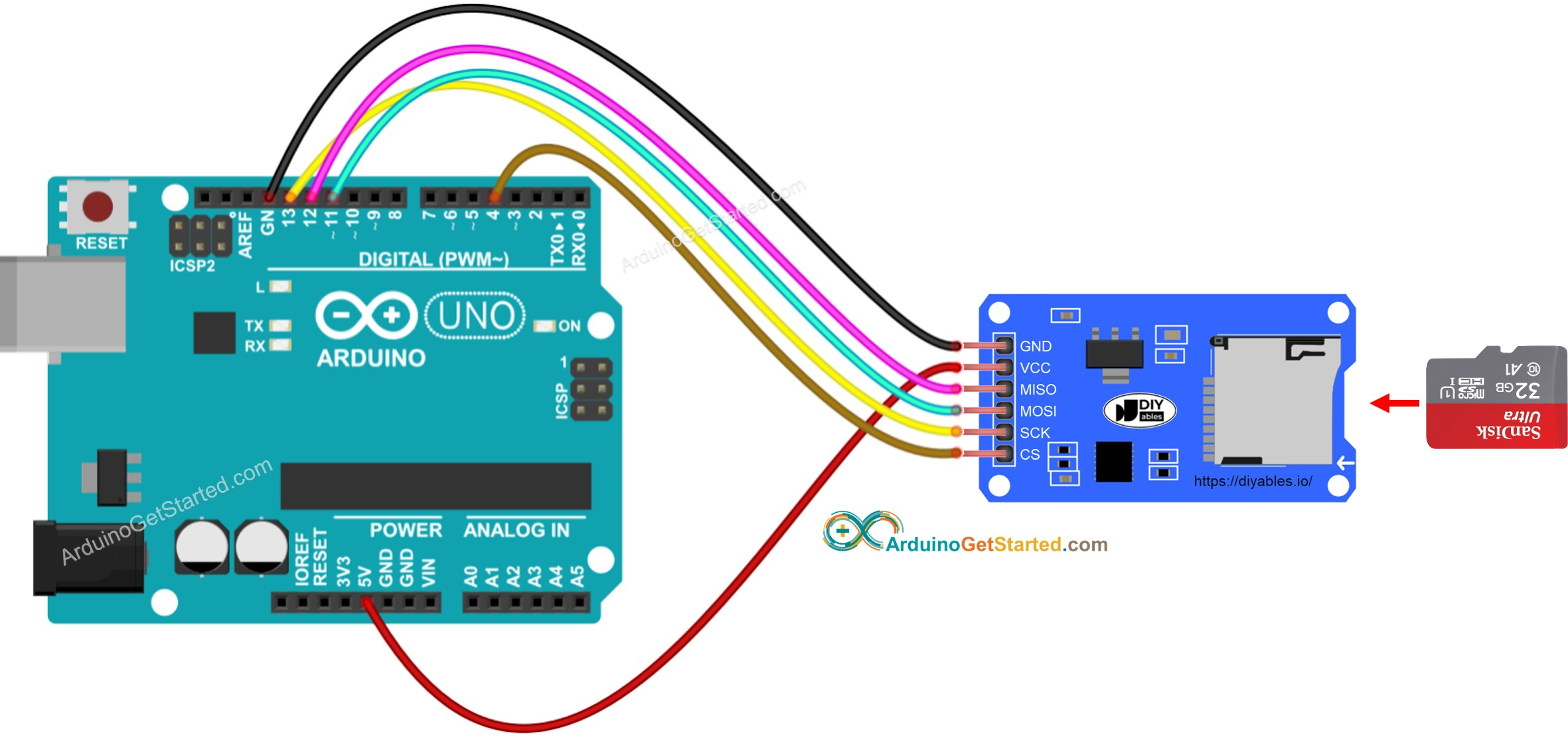# Arduino - Read Config from SD Card

In this tutorial, we are going to learn how to use the config file on Micro SD Card. In detail, we will learn:

• Save a config.txt file on Micro SD Card. The file contains the key-value pairs
• Read the config from the Micro SD Card and save it into int variable
• Read the config from the Micro SD Card and save it into float variable
• Read the config from the Micro SD Card and save it into String variable## Hardware Required

Or you can buy the following sensor kit:

Please note: These are affiliate links. If you buy the components through these links, We may get a commission at no extra cost to you. We appreciate it.

## About Micro SD Card Module

If you do not know about Micro SD Card Module (pinout, how it works, how to program ...), learn about them in the Arduino - Micro SD Card tutorial.

## How It Works

The key-value pairs are pre-stored on the Micro SD Card according to the following format:

• Each key-value pair is on one line. In another word, each key-value pair is separated from others by a newline character
• The key and value are separated by a = character

Arduino code will search the key and find the corresponding value, and save the value to a variable. The variable type can be int, float, or String

## Wiring DiagramThis image is created using Fritzing. Click to enlarge image

※ NOTE THAT:

If you use an Ethernet shield or any shield that has a Micro SD Card Holder, you do not need to use the Micro SD Card Module. You just need to insert the Micro SD Card to the Micro SD Card Holder on the shield.

## How To read config to variables

• Copy below key-value pairs and paste it to the config.txt:
myString_1=Hello myString_2=ArduinoGetStarted.com myInt_1=2 myInt_2=-105 myFloat_1=0.74 myFloat_2=-46.08
• Connect the Micro SD Card to the PC via USB 3.0 SD Card Reader
• Make sure that the Micro SD Card is formatted FAT16 or FAT32 (Google for it)
• Copy config.txt file to the root directory of the Micro SD Card
• Disconnect the Micro SD Card from PC
• Connect the Micro SD Card to Arduino via Micro SD Card Module according to the above wiring diagram
• Copy the below code and open with Arduino IDE
/* * Created by ArduinoGetStarted.com * * This example code is in the public domain * * Tutorial page: https://arduinogetstarted.com/tutorials/arduino-read-config-from-sd-card */ #include <SD.h> #define PIN_SPI_CS 4 #define FILE_NAME "config.txt" #define KEY_MAX_LENGTH 30 // change it if key is longer #define VALUE_MAX_LENGTH 30 // change it if value is longer // variables int myInt_1; int myInt_2; float myFloat_1; float myFloat_2; String myString_1; String myString_2; void setup() { Serial.begin(9600); if (!SD.begin(PIN_SPI_CS)) { Serial.println(F("SD Card failed, or not present")); while (1); // don't do anything more: } Serial.println(F("SD Card initialized.")); myInt_1 = SD_findInt(F("myInt_1")); myInt_2 = SD_findInt(F("myInt_2")); myFloat_1 = SD_findFloat(F("myFloat_1")); myFloat_2 = SD_findFloat(F("myFloat_2")); myString_1 = SD_findString(F("myString_1")); myString_2 = SD_findString(F("myString_2")); Serial.print(F("myInt_1 = ")); Serial.println(myInt_1); Serial.print(F("myInt_2 = ")); Serial.println(myInt_2); Serial.print(F("myFloat_1 = ")); Serial.println(myFloat_1); Serial.print(F("myFloat_2 = ")); Serial.println(myFloat_2); Serial.print(F("myString_1 = ")); Serial.println(myString_1); Serial.print(F("myString_2 = ")); Serial.println(myString_2); } void loop() { } bool SD_available(const __FlashStringHelper * key) { char value_string[VALUE_MAX_LENGTH]; int value_length = SD_findKey(key, value_string); return value_length > 0; } int SD_findInt(const __FlashStringHelper * key) { char value_string[VALUE_MAX_LENGTH]; int value_length = SD_findKey(key, value_string); return HELPER_ascii2Int(value_string, value_length); } float SD_findFloat(const __FlashStringHelper * key) { char value_string[VALUE_MAX_LENGTH]; int value_length = SD_findKey(key, value_string); return HELPER_ascii2Float(value_string, value_length); } String SD_findString(const __FlashStringHelper * key) { char value_string[VALUE_MAX_LENGTH]; int value_length = SD_findKey(key, value_string); return HELPER_ascii2String(value_string, value_length); } int SD_findKey(const __FlashStringHelper * key, char * value) { File configFile = SD.open(FILE_NAME); if (!configFile) { Serial.print(F("SD Card: error on opening file ")); Serial.println(FILE_NAME); return; } char key_string[KEY_MAX_LENGTH]; char SD_buffer[KEY_MAX_LENGTH + VALUE_MAX_LENGTH + 1]; // 1 is = character int key_length = 0; int value_length = 0; // Flash string to string PGM_P keyPoiter; keyPoiter = reinterpret_cast<PGM_P>(key); byte ch; do { ch = pgm_read_byte(keyPoiter++); if (ch != 0) key_string[key_length++] = ch; } while (ch != 0); // check line by line while (configFile.available()) { int buffer_length = configFile.readBytesUntil('\n', SD_buffer, 100); if (SD_buffer[buffer_length - 1] == '\r') buffer_length--; // trim the \r if (buffer_length > (key_length + 1)) { // 1 is = character if (memcmp(SD_buffer, key_string, key_length) == 0) { // equal if (SD_buffer[key_length] == '=') { value_length = buffer_length - key_length - 1; memcpy(value, SD_buffer + key_length + 1, value_length); break; } } } } configFile.close(); // close the file return value_length; } int HELPER_ascii2Int(char *ascii, int length) { int sign = 1; int number = 0; for (int i = 0; i < length; i++) { char c = *(ascii + i); if (i == 0 && c == '-') sign = -1; else { if (c >= '0' && c <= '9') number = number * 10 + (c - '0'); } } return number * sign; } float HELPER_ascii2Float(char *ascii, int length) { int sign = 1; int decimalPlace = 0; float number = 0; float decimal = 0; for (int i = 0; i < length; i++) { char c = *(ascii + i); if (i == 0 && c == '-') sign = -1; else { if (c == '.') decimalPlace = 1; else if (c >= '0' && c <= '9') { if (!decimalPlace) number = number * 10 + (c - '0'); else { decimal += ((float)(c - '0') / pow(10.0, decimalPlace)); decimalPlace++; } } } } return (number + decimal) * sign; } String HELPER_ascii2String(char *ascii, int length) { String str; str.reserve(length); str = ""; for (int i = 0; i < length; i++) { char c = *(ascii + i); str += String(c); } return str; }
• Click Upload button on Arduino IDE to upload code to Arduino
• See the result on Serial Monitor.
COM6
SD Card initialized. myInt_1 = 2 myInt_2 = -105 myFloat_1 = 0.74 myFloat_2 = -46.08 myString_1 = Hello myString_2 = ArduinoGetStarted.com
Autoscroll Show timestamp
Clear output
9600 baud
Newline
• The Serial Monitor shows the values from variables that is identical to the values saved on the micro SD Card

Now you can modify the code to add more variables.

※ NOTE THAT:

The above code does not care about the order of key-value pairs. It will search for the key from the beginning to the end of the file until the key is matched.

## Video Tutorial

We are considering to make the video tutorials. If you think the video tutorials are essential, please subscribe to our YouTube channel to give us motivation for making the videos.